11. DUAL NATURE OF MATTER AND RADIATION

Q.1. What is the photoelectric effect and how did Einstein explain it ?

Ans ⇒ Photoelectric effect : The phenomenon of emission of electrons from a metal surface, when light of sufficiently high frequency falls upon it, is called photoelectric effect. It is initiated by Hallwach.
The electron so emitted were called photo electrons. The current constituted by photoelectrons is called photoelectric current.
J.J. Thomson informed that photo electrons were not different from ordinary electrons.
In 1905 Einstein explained the phenomena on the basis of quantum theory given by Plank. According to quantum theory of light energy is bundle of smallpackets of energy called quantum or photon. Its energy, E = hy, where is frequency of photon and h is Planck’s constant.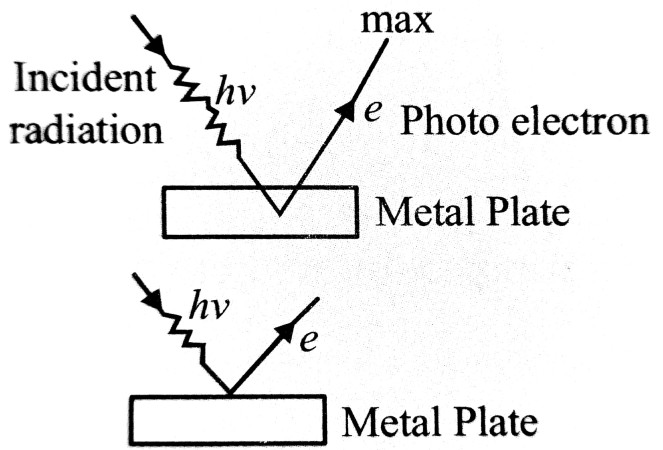Photo-electric equation or photo-Einstein equation is given by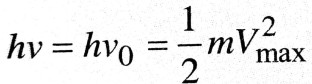Here, → hv + energy of incident photon,
hv0 → energy of incident photon,
hv0 → work function where v0 is
threshold frequency of photon &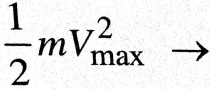kinetic energy (K. E.) of emitted electrons.
When a photon is incident on a metal plate its total energy is absorbed by an electron in plate. Some
part of energy received by electron is spent in coming out the metal or over coming surface barrier. Remaining enegy in electron is in the form of K. E. of electron. Since electrons from metal plates are emitted from different layers so they have different amount of energy. Electron during its journey in metals strikes ions and neutral atoms. So they lose some part of its energy. So electrons rejected from surface of metal has maximum K. E.

If V > v0 thens electron comes out metal with some amount of K. E.
If v = v0 then emission of electrons.
If v < V0 then electrons are not emitted takes place.
Photo-electric current depends upon intensity of incident radiation but K.E. of emitted electron depends upon frequency of incident photon.

Q.2. Derive Einstein’s mass energy relation E = mc2.

Ans ⇒ By the special theory of relativity the mass of a body moving with velocity v is
.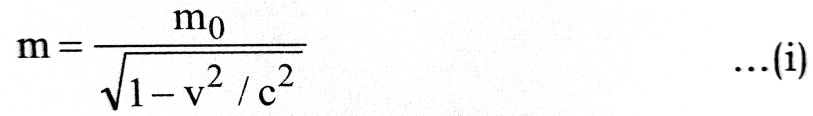where m0 = rest mass and C is the speed of light.
According to Classical mechanics, rate of change of momentum is equal to force i.e.
.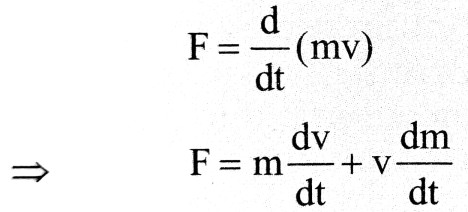Let due to application of force F, the body is displaced by distance dx. The charge in kinetic energy dT is equal to the work done.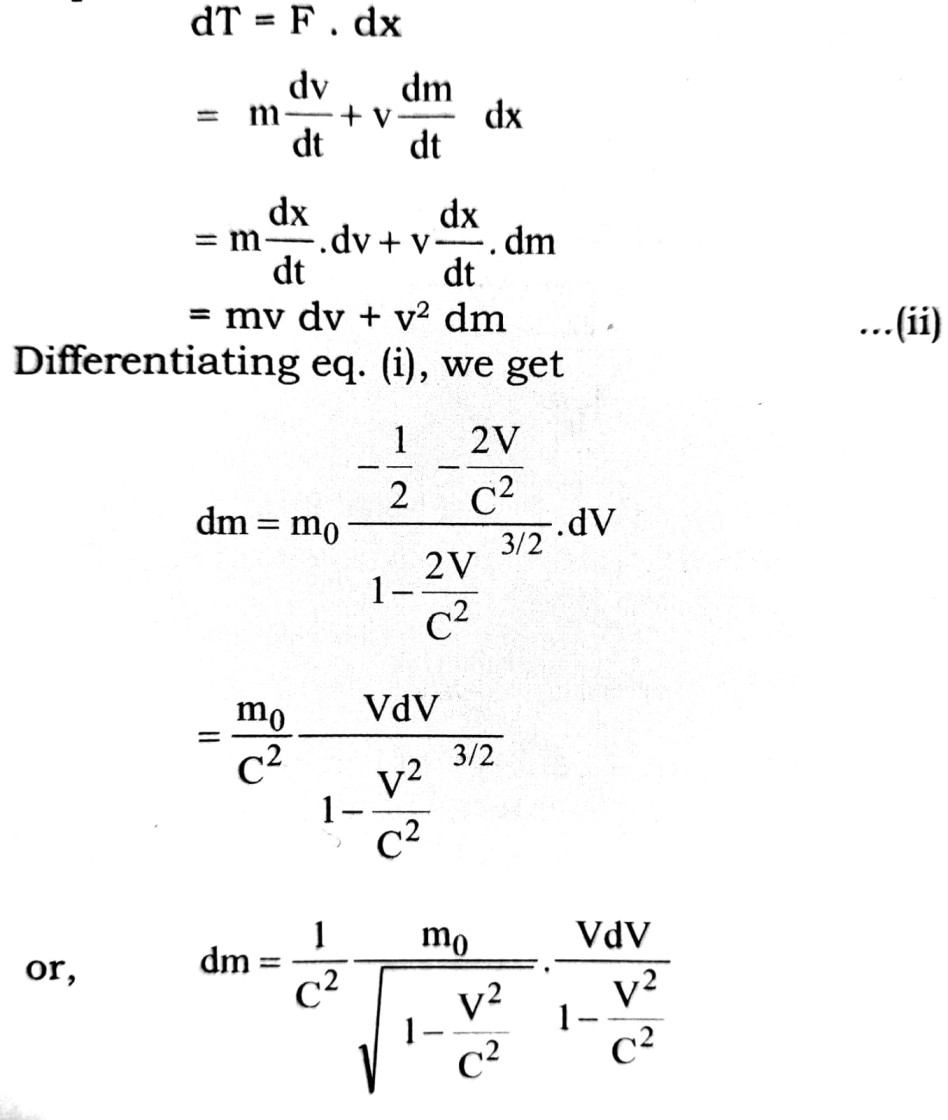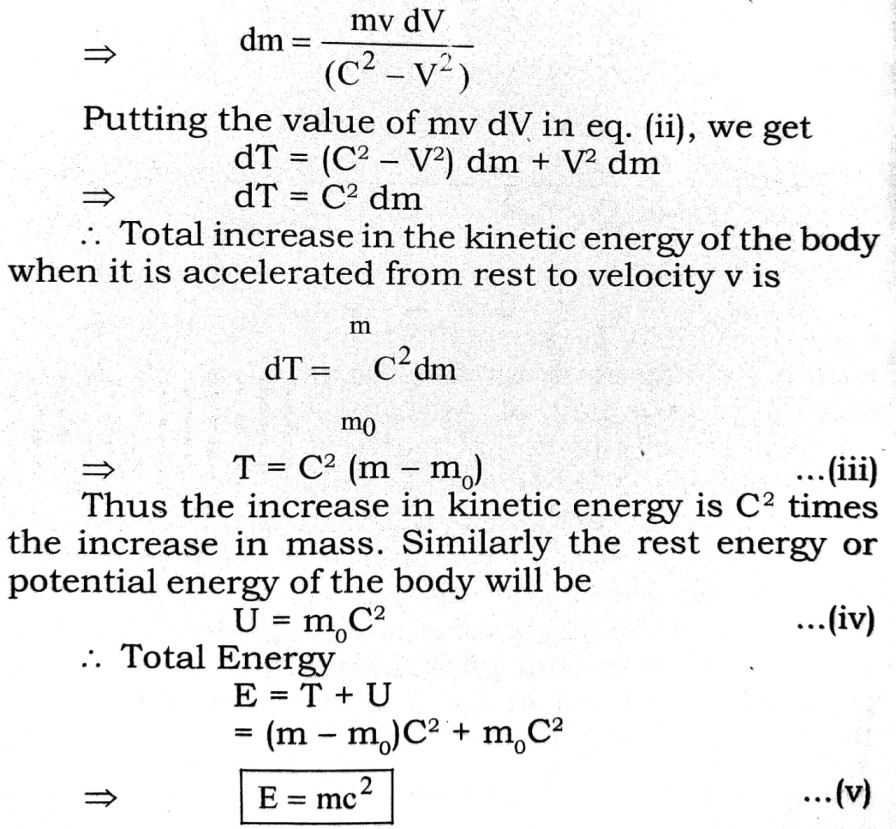Q.3. What is meant by dual nature of matter ? Derive an expression for the wavelength of a matter wave established by de Broglie.

Ans ⇒ On the basis of wave theory of light many optical phenomena like interference, diffraction, polarisation have been explained successfully. But some optical phenoman like photo electric effect, Crompton effect could not been explained on the wave theory. These phenomena have been explained by the Quantum theory enuciated by max Planck. According to Quatum theory the emission or absorption of light is not continuous but discrete i.e., light energy propagates in the form of small pockets of energy which are called photon. If the frequency of light is v then the energy of light E = hv, where his a constant called Planck’s constant. In this way for the explanation of all optical phenomena wave theory and Quantum theory both are essential. Hence it has been established that the nature of light is Dual i.e., both properties photon and wave are found in light.
On the observation of dual nature of light French scientist Lousie de Broglie proposed that the dual nature is found not only in light but material particles also. According to him when a material particle like electron or photon moves then it behaves like a wave. These waves are known as matter waves or de Broglie waves.
Wavelength of matter waves :
Let the frequency of light wae is v then the energy of photon
E = hv                           … (i)
where his Planck’s constant.
If photon is supposed to be a particle of mass m then according to Eistein’s special theory of relatively.
E = mc2                       … (ii)
where c is the velocity of light.
From eq. (i) and (ii),
.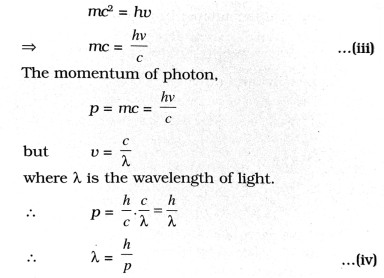If a particle of mass m moves with velocity v, then its momentum
p = mv
Hence the wavelength linked with the particle is
.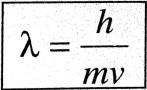…(v)

In this way the wavelength linked with the wave of a moving particle depends upon its momentum.

## Class 12th physics Long Type question in English

 S.N Physics Long Type Question English Medium 1. ELECTRIC CHARGES AND FIELDS 2. LECTROSTATIC POTENTIAL AND CAPACITANCE 3. 4. 5. MAGNETISM AND MATTER 6. ELECTROMAGNETIC INDUCTION 7. ALTERNATING CURRENT 8. ELECTROMAGNETIC WAVES 9. RAY OPTICS AND OPTICAL INSTRUMENTS 10. WAVE OPTICS 11. DUAL NATURE OF MATTER AND RADIATION 12. ATOMS 13. NUCLEI 14. SEMI CONDUCTOR ELECTRONICS 15. COMMUNICATION SYSTEMS
You might also like